# RD Sharma Class 12 Solutions Chapter 5 Algebra of Matrices Ex 5.4

RD Sharma Class 12 Solutions Chapter 5 Algebra of Matrices Ex 5.4 are part of RD Sharma Class 12 Solutions. Here we have given RD Sharma Class 12 Solutions Chapter 5 Algebra of Matrices Ex 5.4.

Here you can get free RD Sharma Solutions for Class 12 Maths of Chapter 5 Algebra of Matrices Exercise 5.4. All RD Sharma Book Solutions are given here exercise wise for Algebra of Matrices. RD Sharma Solutions are helpful in the preparation of several school level, graduate and undergraduate level competitive exams. Practicing questions from RD Sharma Mathematics Solutions for Class 12 Chapter 5 Algebra of Matrices is proven to enhance your math skills.

 Class: 12th Class Chapter: Chapter 5 Name: Algebra of Matrices Exercise: Exercise 5.4

## RD Sharma Class 12 Solutions Chapter 5 Algebra of Matrices Ex 5.4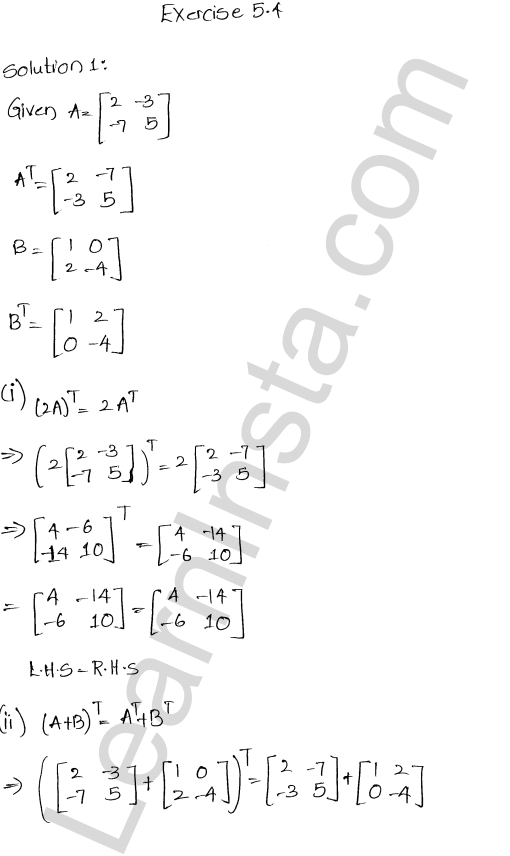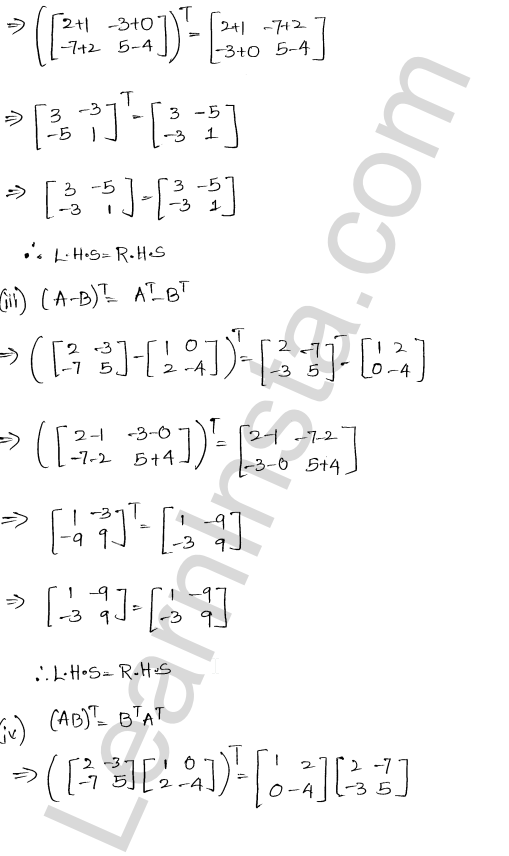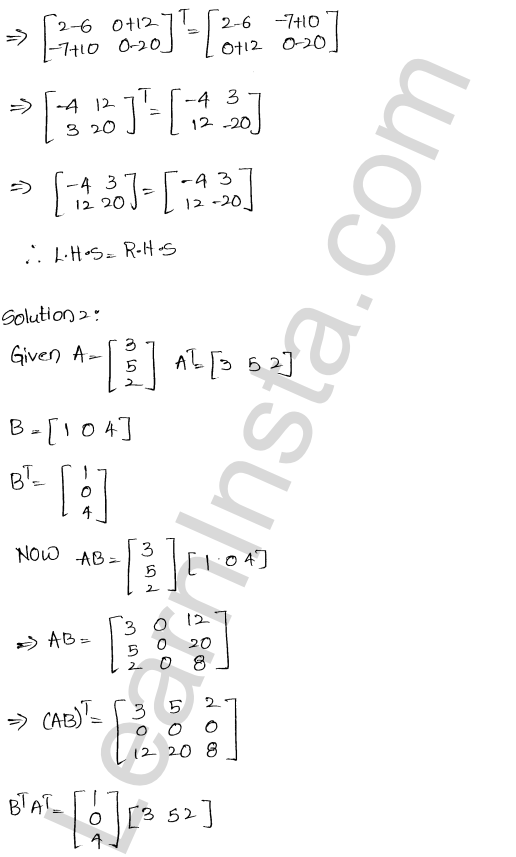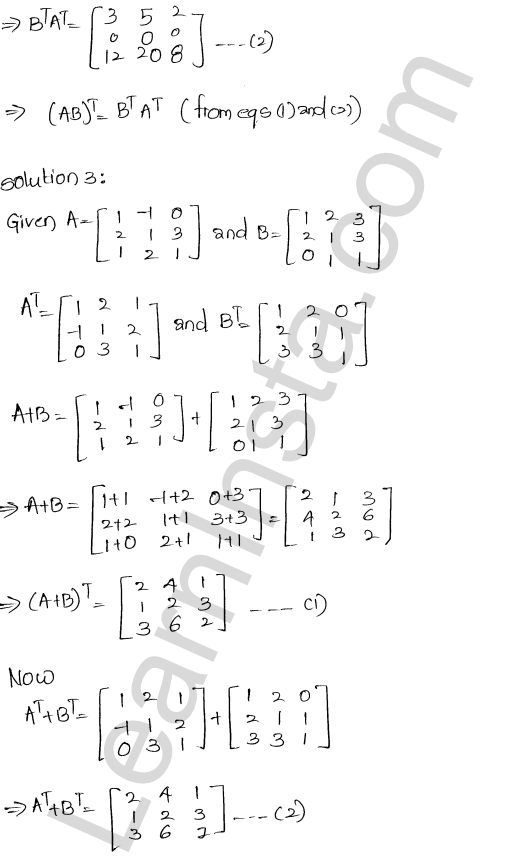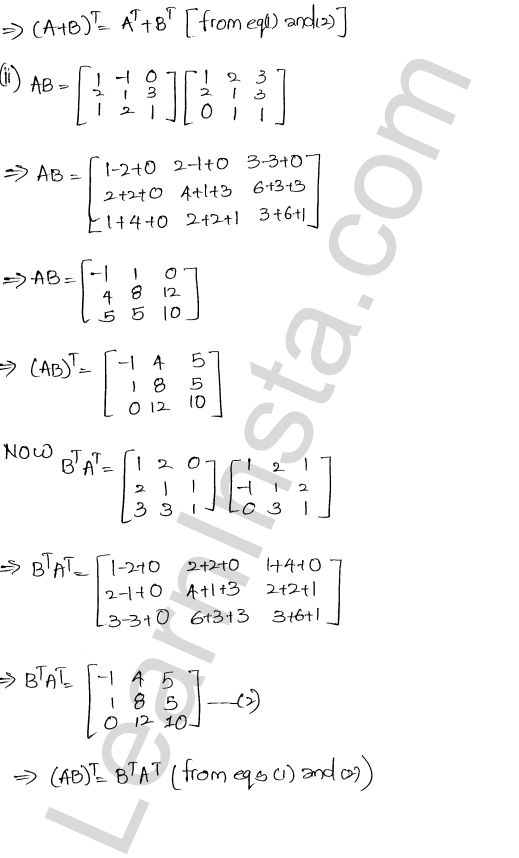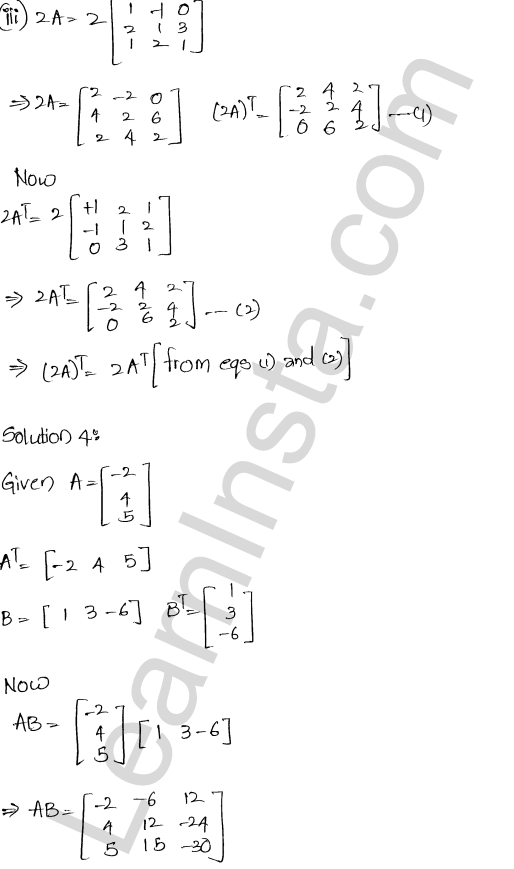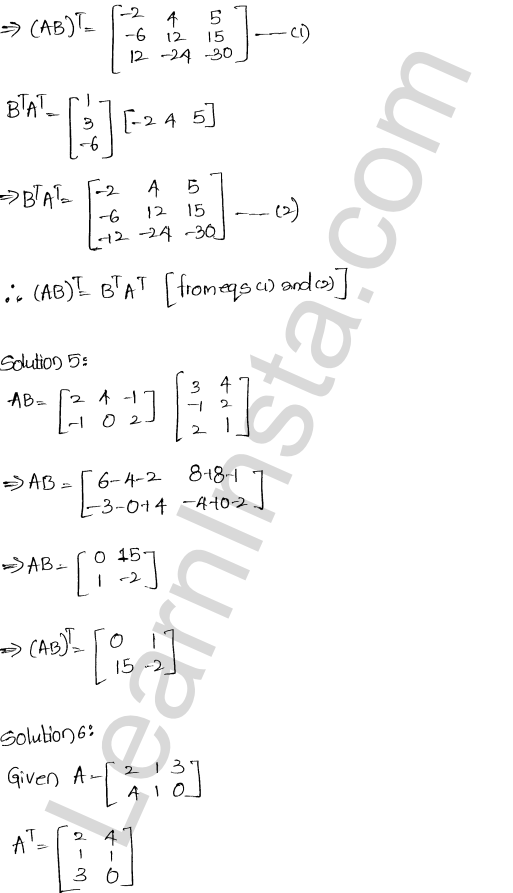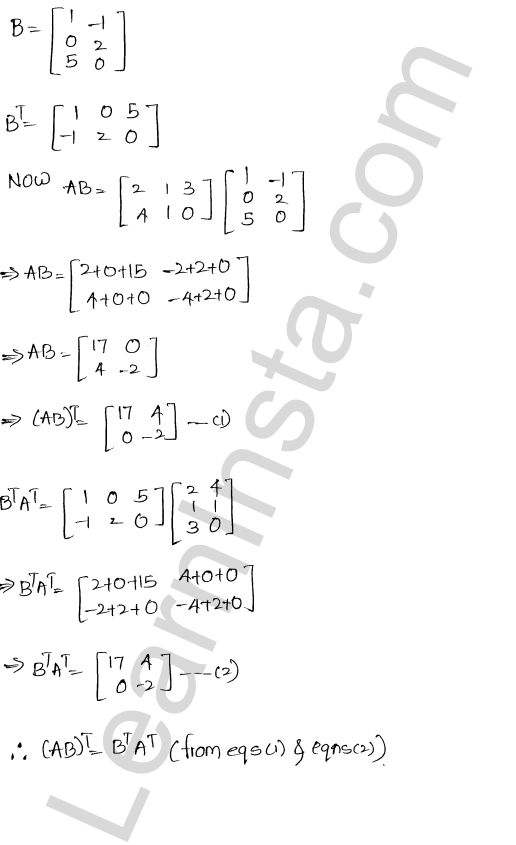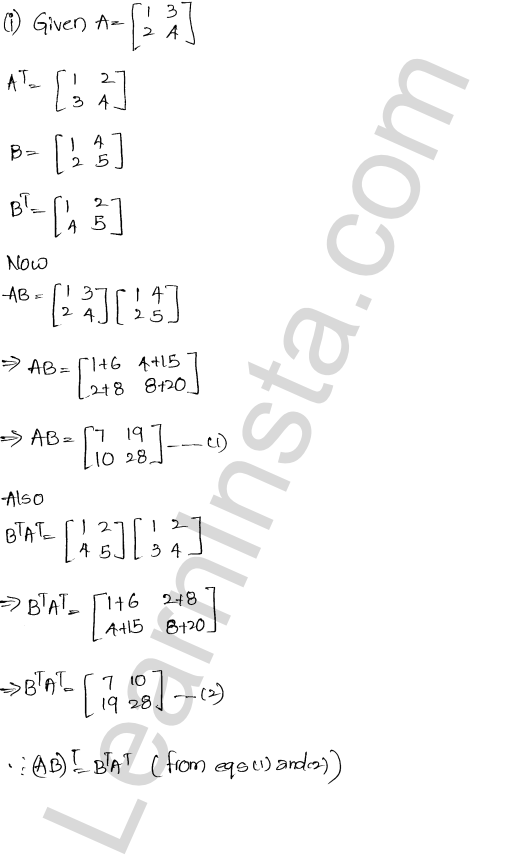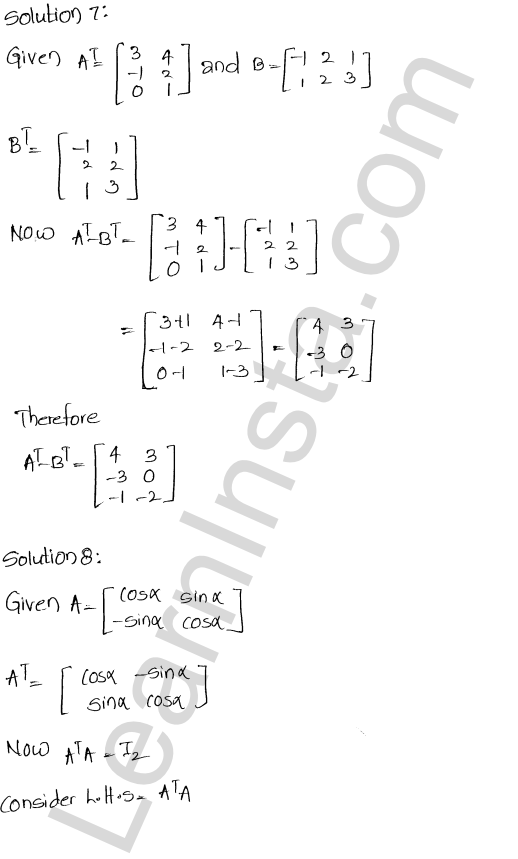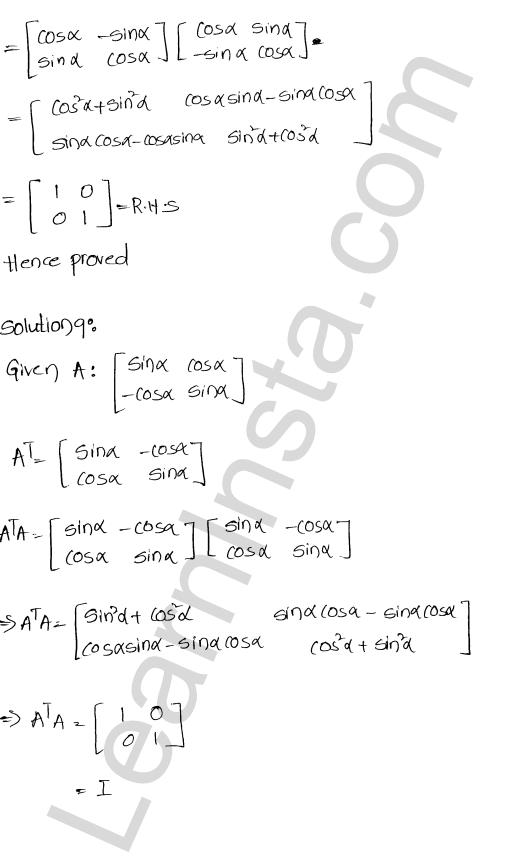We hope the RD Sharma Class 12 Solutions Chapter 5 Algebra of Matrices Ex 5.4, help you. If you have any query regarding RD Sharma Class 12 Solutions Chapter 5 Algebra of Matrices Ex 5.4, drop a comment below and we will get back to you at the